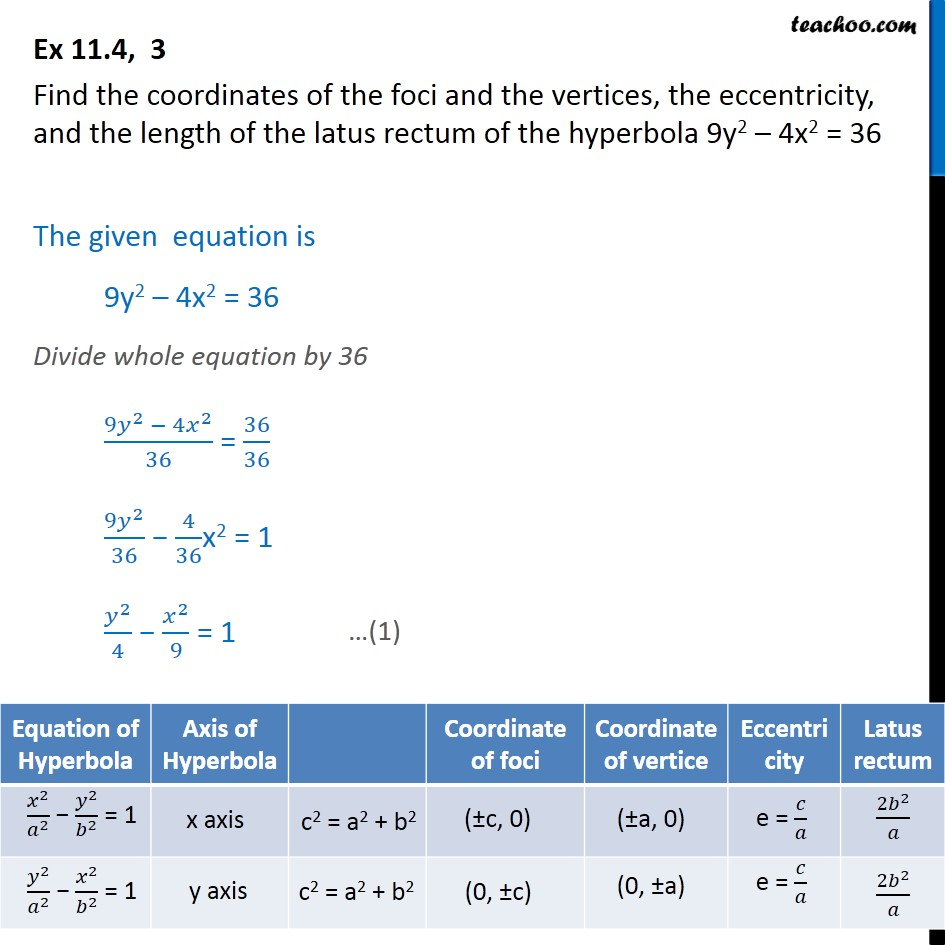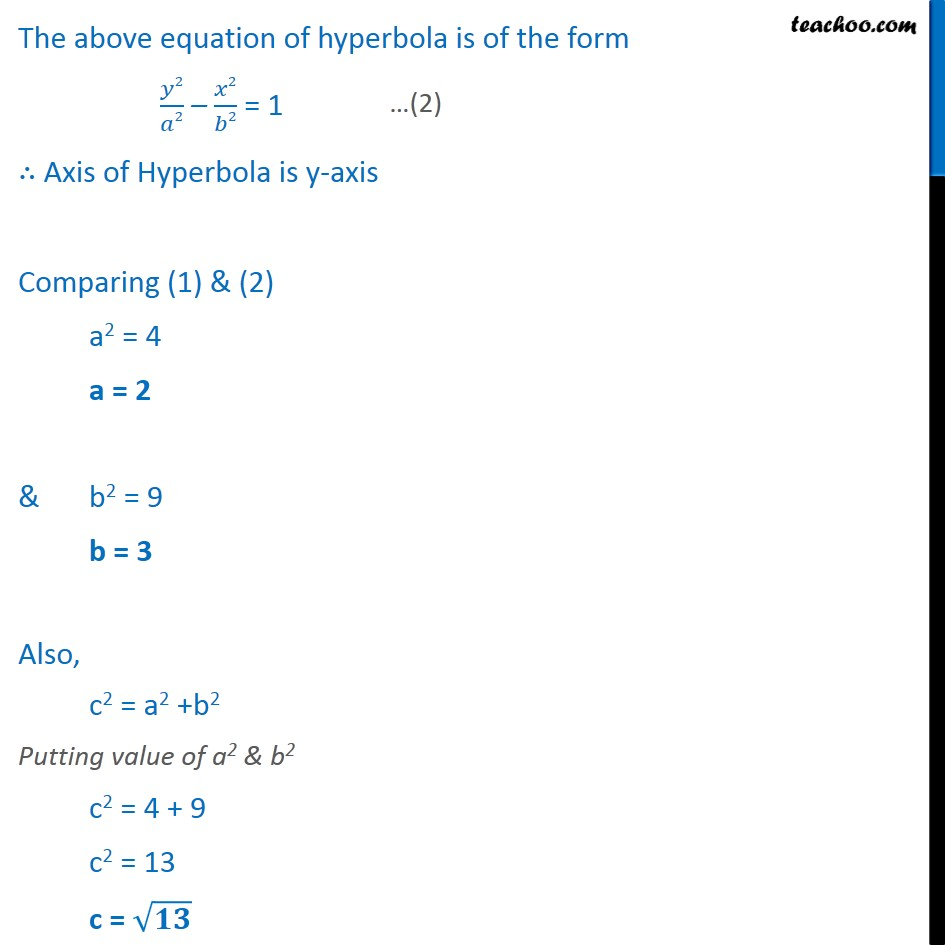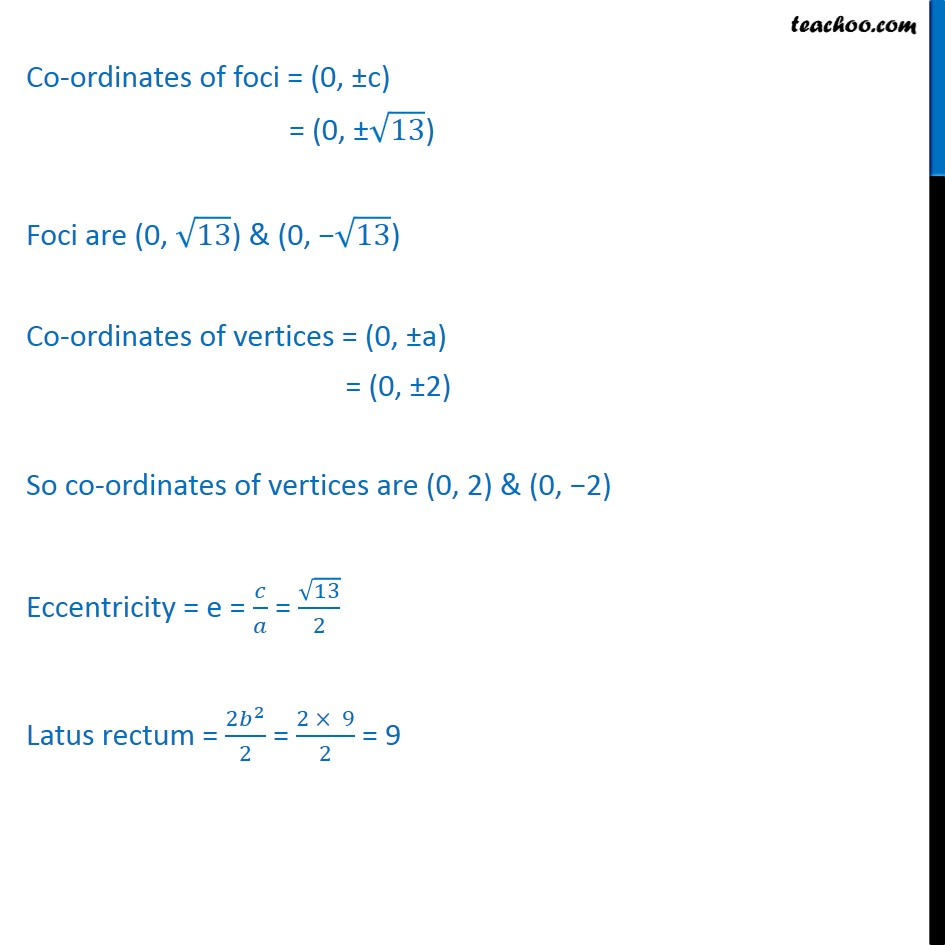Ex 10.4

Chapter 10 Class 11 Conic Sections
Serial order wiseLearn in your speed, with individual attention - Teachoo Maths 1-on-1 Class

### Transcript

Ex 10.4, 3 Find the coordinates of the foci and the vertices, the eccentricity, and the length of the latus rectum of the hyperbola 9y2 – 4x2 = 36 The given equation is 9y2 – 4x2 = 36 Divide whole equation by 36 ﷐9﷐𝑦﷮2﷯ − 4﷐𝑥﷮2﷯﷮36﷯ = ﷐36﷮36﷯ ﷐9﷐𝑦﷮2﷯﷮36﷯ − ﷐4﷮36﷯x2 = 1 ﷐﷐𝑦﷮2﷯﷮4﷯ − ﷐﷐𝑥﷮2﷯﷮9﷯ = 1 The above equation of hyperbola is of the form ﷐𝑦2﷮𝑎2﷯ – ﷐𝑥2﷮𝑏2﷯ = 1 ∴ Axis of Hyperbola is y-axis Comparing (1) & (2) a2 = 4 a = 2 & b2 = 9 b = 3 Also, c2 = a2 +b2 Putting value of a2 & b2 c2 = 4 + 9 c2 = 13 c = ﷐﷮𝟏𝟑﷯ Co-ordinates of foci = (0, ±c) = (0, ±﷐﷮13﷯) Foci are (0, ﷐﷮13﷯) & (0, −﷐﷮13﷯) Co-ordinates of vertices = (0, ±a) = (0, ±2) So co-ordinates of vertices are (0, 2) & (0, −2) Eccentricity = e = ﷐𝑐﷮𝑎﷯ = ﷐﷐﷮13﷯﷮2﷯ Latus rectum = ﷐2﷐𝑏﷮2﷯﷮2﷯ = ﷐2 × 9﷮2﷯ = 9